# An LCR circuit is equivalent to a damped pendulum. In an LCR circuit the capacitor is charged to Q0 and then connected to the L and R as shown below :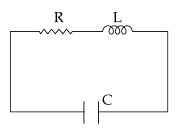If a student plots graphs of the square of maximum charge ( Q2Max ) on the capacitor with time(t) for two different values L1 and L2 (L 1>L2) of L then which of the following represents this graph correctly ? (plots are schematic and not drawn to scale) Option 1)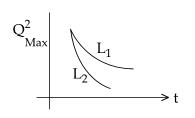Option 2)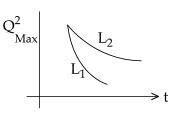Option 3)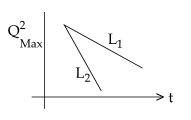Option 4)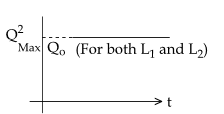As we learnt in

LR Circuit Voltage -

- wherein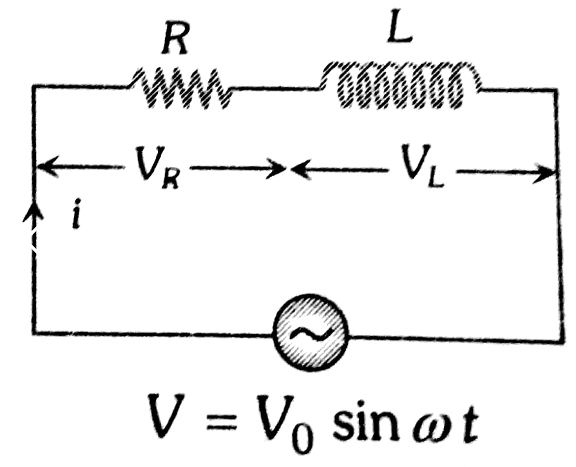damping constant for a simple pendulum is inversely proportional to m.

Here damping constant is inversely proportional to L.

second case will have higher rate of decay & the graph will follow the exponential equation.

Option 1)This option is correct.

Option 2)This option is incorrect.

Option 3)This option is incorrect.

Option 4)This option is incorrect.

### Preparation Products

##### JEE Main Rank Booster 2021

This course will help student to be better prepared and study in the right direction for JEE Main..

₹ 13999/- ₹ 9999/-
##### Knockout JEE Main April 2021 (Subscription)

An exhaustive E-learning program for the complete preparation of JEE Main..

₹ 4999/-
##### Knockout JEE Main April 2021

An exhaustive E-learning program for the complete preparation of JEE Main..

₹ 22999/- ₹ 14999/-
##### Knockout JEE Main April 2022

An exhaustive E-learning program for the complete preparation of JEE Main..

₹ 34999/- ₹ 24999/-